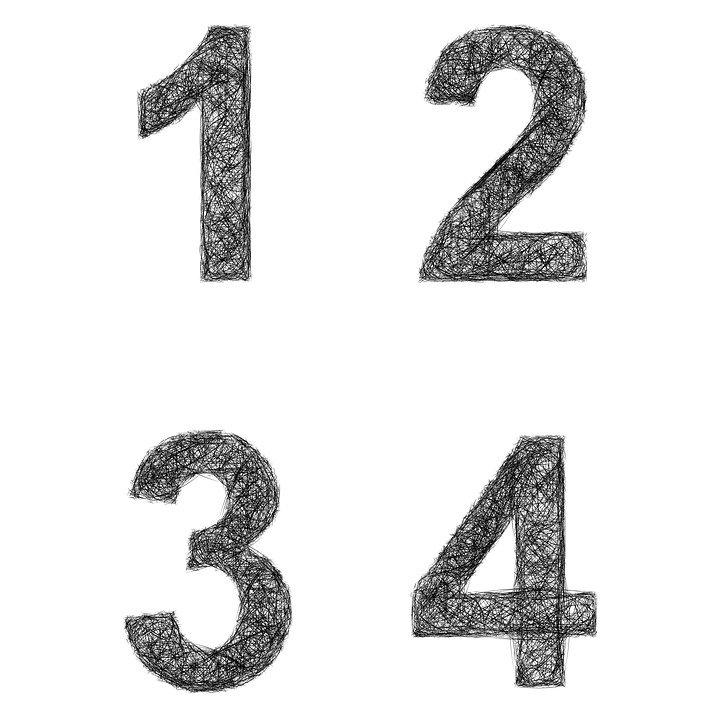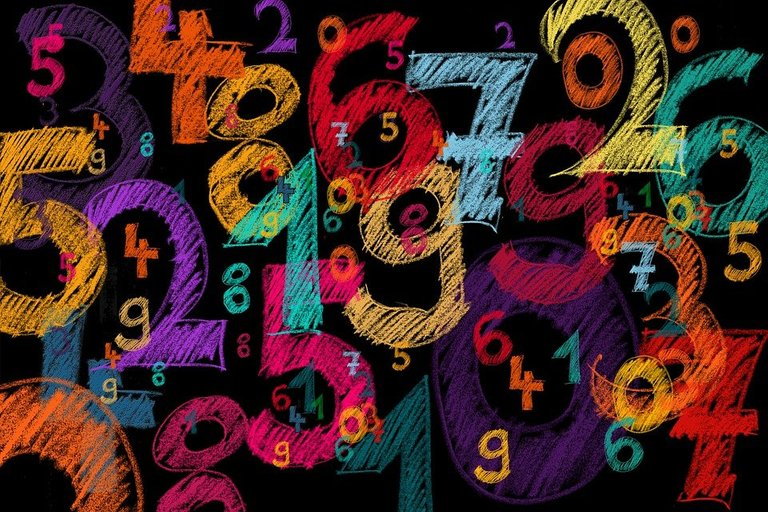# Divisibility Rule For 17

in STEMGeekslast year

Hi there. In this short math post, I cover the divisibility rule for 17. This rule can be helpful if a calculator is not allowed and if long division is not your thing.Pixabay Image Source

## The Procedure

It is best to show if a large number can be divided by 17 through examples.

Example One

Is 121 divisible by 17?

• The last digit in 121 is the `1` in the ones place.
• The digits that are not in the ones place are the 1 and 2 that make `12`.

Use this 12 subtracted by five times the ones place digit. That is 12 - 5 = 7. Is this seven divisible by 7? If yes, then 121 is divisible by 17. If not, then 121 is not divisible by 17.

As 7 is not divisible by 17, the conclusion is that 121 is not divisible by 17.

Note that the multiplying five with the last digit is part of the procedure. (Why? I do not know, it just works.)Pixabay Image Source

Example Two

Let's work with a larger number such as 1234. Is 1234 divisible by 17?

• The last digit is a 4 with the other digits being 123 to form `123`.
• Do 123 minus `4 x 5`. This would be 123 minus 20 to obtain 103.
• Is 103 divisible by 17? It can be hard to say. Let's repeat the procedure.

• The new last digit is 3 from 103 with the other digits forming 10.
• Calculate 10 minus 3 times 5 gives `10 minus 15` which is negative 5.

Negative 5 is not divisible by 17, so 1234 is not divisible by 17.

Example Three

Is 2618 divisible by 17?

The last digit in 2618 is 8. Multiply this 8 by 5 to obtain 40.

The other digits form the number 261. Use 261 minus 40 to obtain 221. It is not obvious if 221 is divisible by 17. Run the procedure again on 221.

In 221, the last digit is 1. Run 22 minus 5 times 1 which is 22 minus 5 which is 17. Seventeen is divisible by 17 which makes 2618 divisible by 17.Pixabay Image Source

Posted with STEMGeeks

Sort:
last year

Haha wow, this is like one of the most complex rules I’ve seen to date.

This one is kind of hard to remember as you won't use this often lol.

last year

This is one of those random extra credit thing in math class I feel.